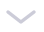## Take you to brush (Nioke.com) C language 100 questions (tenth day)

✅Author's introduction: Hello everyone, I am @I have to type code every day, a player who is a material transfer coder, I hope to work hard and make progress together!
📃Personal homepage: @The personal homepage who has to type codes every
day🔥Series of columns: Niuke.com brush questions column
💬Recommend a mock interview, brush questions artifact, from basic to big factory interview questions 👉Click to jump to the brush questions website to register studyExercise 1: The Four Seasons

Exercise 2: Health Assessment

Exercise 3: Xiaolele finds the largest number

Exercise 4: Judging whether it is a letter

Exercise 5: Divide by 237 of Niuniu

Exercise 6: Positive and Negative Numbers of Statistical Data

concluding remarks

## describe

Meteorologically, spring is usually from March to May, summer is from June to August, autumn is from September to November, and winter is from December to February. Please output the corresponding season according to the input year and month.

### Enter description:

The input data format is a fixed YYYYMM format, that is, the year occupies 4 digits, and the month occupies 2 digits.

### Output description:

Output the season corresponding to the month (expressed in English words, all in lowercase).

## Example 1

Input: 201901

output: winter

Note: The year entered should be 4 digits, and the month entered should be 1~12.

``#include<stdio.h>int main(){    int year,month;    scanf("%4d%2d", &year, &month);    if(month >= 3 && month <= 5)        printf("spring\n");    else if(month >= 6 && month <= 8)        printf("summer\n");    else if(month >= 9 && month <= 11)        printf("autumn\n");    else        printf("winter\n");    return 0;}``

## describe

The BMI index (ie body mass index) is a number obtained by dividing the weight in kilograms by the square of the height in meters. For example: a person is 1.75 meters tall and weighs 68 kg, his BMI=68/(1.75^2)=22.2 (kg/m^2). When the BMI index is 18.5 to 23.9, it is normal, otherwise it means that the body has a health risk. Program to judge human health.

### Enter description:

On one line, enter a person's weight (kg) and height (m), separated by a space.

### Output description:

One line, output body Normal (normal) or Abnormal (abnormal).

Input: 68 1.75

Output: Normal

## Example 2

Input: 67.5 1.65

Output: Abnormal

``#include <stdio.h>int main(){    float w,h;    scanf("%f %f",&w,&h);    float BMI=w/(h*h);    if(BMI>=18.5 && BMI<=23.9)        printf("Normal");    else        printf("Abnormal");    return 0;}``

## describe

### Enter description:

One line, 4 integers, separated by spaces.

### Output description:

A line, an integer, is the largest integer among the four input integers.

## Example 1

Input: 5 8 2 5

output: 8

``#include <stdio.h>int main(){    // method 1    int a,b,c,d;    scanf("%d%d%d%d",&a,&b,&c,&d);    if(a<b)    {        int tmp=a;        a=b;        b=tmp;    }    if(a<c)    {        int tmp=a;        a=c;        c=tmp;    }    if(a<d)    {        int tmp=a;        a=d;        d=tmp;    }    printf("%d",a);     // method 2 int main(){    int arr={0};    for(int i =0;i<4;i++)        scanf("%d",&arr[i]);    int max = arr;    for(int i=0;i<4;i++){        if(max<arr[i]){            max = arr[i];        }    }    printf("%d\n",max);    return 0;}``

## describe

KiKi wants to determine whether the input character is a letter or not, please help him program it.

### Enter description:

Multiple sets of input, one character per line.

### Output description:

For each group of input, the output occupies a separate line to determine whether the input character is a letter. For the output content, see the output example.

## Example 1

enter:

A

6

output:

A is an alphabet.

6 is not an alphabet.

``#include <stdio.h>int main(){    int ch=0;    while((ch=getchar()) != EOF)    {        if((ch>='A' && ch<='Z') || (ch>='a' && ch<='z'))        {            printf("%c is an alphabet.\n",ch);             getchar();        }        else        {            printf("%c is not an alphabet.\n",ch);            getchar();        }    }        return 0;}``

## describe

Niu Niu input an integer from the keyboard, please judge which number of 2 3 7 this integer can be divided by, and output it in ascending order. If it is not divisible by any number 2 3 7 then output n.

enter an integer

### Output description:

Output which numbers are divisible by 2 3 7 and output in ascending order.

Input: 6

Output: 2 3

Input: 3

output: 3

Input: 14

Output: 2 7

## Example 4

Input: 11

output: n

``#include <stdio.h>int main(){    int n=0;    scanf("%d",&n);    if(n%2==0)        printf("2 ");    if(n%3==0)        printf("3 ");    if(n%7==0)        printf("7 ");    if(n%2!=0 && n%3!=0 && n%7!=0)        printf("n ");    return 0;}``

## describe

Input 10 integers, and output the number of positive and negative numbers respectively.

### Enter description:

Enter 10 integers (ranges ), separated by spaces.

### Output description:

Two lines, the first line contains positive numbers, and the second line contains negative numbers. See the example for the specific format.

## Example 1

Input: -1 2 3 -6 7 8 -1 6 8 10

output:

positive:7

negative:3

``#include <stdio.h>int main(){    int arr={0};    int i=0;    int count1=0,count2=0;    for(i=0;i<10;i++)    {        scanf("%d ",&arr[i]);    }    for(i=0;i<10;i++)    {    if(arr[i]>0)        count1++;    else        count2++;    }    printf("positive:%d\n",count1++);    printf("negative:%d",count2++);        return 0;}``

# concluding remarks

Today's sharing is here!

Sign up to join the quiz army through the link below! The real interview questions of various big factories are waiting for you!#### Tags: Take you to brush (Nioke.com) C language 100 questions (tenth day)

Niuke.com C language brushing column c language algorithm Development language